# NCERT Solutions (Part - 1) - Simple Equations Class 7 Notes | EduRev

## Class 7 : NCERT Solutions (Part - 1) - Simple Equations Class 7 Notes | EduRev

The document NCERT Solutions (Part - 1) - Simple Equations Class 7 Notes | EduRev is a part of the Class 7 Course Mathematics (Maths) Class 7.
All you need of Class 7 at this link: Class 7

Exercise 4.1
Ques 1: Complete the last column of the table:

 S. No. Equation Value Say, whether the Equation is satisfied. (Yes / No) (i) x + 3 = 0 x = 3 (ii) x + 3 = 0 x = 0 (iii) x + 3 = 0 x = -3 (iv) x - 7 = 1 x = 7 (v) x - 7 = 1 x = 8 (vi) 5x = 25 x = 0 (vii) 5x = 25 x = 5 (viii) 5x = 25 x = -5 (viii) m/3 = 2 w = -6 (ix) m/3 = 2 m = 0 (x) m/3 = 2 m = 6

 S. No. Equation Value Say, whether the Equation is satisfied. (Yes / No) (i) x + 3 = 0 x = 3 No (ii) x + 3 = 0 x = 0 No (iii) x + 3 = 0 x = -3 Yes (iv) x-7=1 x = 7 No (v) x-7 = 1 x = 8 Yes (vi) 5x = 25 x = 0 No (vii) 5x = 25 x = 5 Yes (viii) 5x = 25 x=-5 No (viii) m/3 = 2 m = -6 No (ix) m/3 = 2 m = 0 No (X) m/3 = 2 m = 6 Yes

Ques 2: Check whether the value given in the brackets is a solution to the given equation or not:
(a) n + 5 = 19(n = 1)
(b) 7n + 5 = 19(n = -2)
(c) 7n + 5 = 19(n = 2)
(d) 4p - 3 = 13(p = 1)
(e) 4p - 3 = 13(p = -4)
(f) 4p - 3 = 13(p = 0)
Ans: (a) n + 5 = 19 (n = 1)
Putting n = 1 in L.H.S.,
1 + 5 = 6
∵ L.H.S. ≠ R.H.S.,
∴ n = 1 is not the solution of given equation.
(b) 7n + 5 = 19(n = -2)
Putting n = -2 in L.H.S.,
7(-2) + 5 = -14 + 5 = -9
∵ L.H.S. ≠ R.H.S.,
∴ n = -2 is not the solution of given equation.
(c) 7n + 5 = 19(n = 2)
Putting n = 2 in L.H.S.,
7(2) + 5 = 14 + 5 = 19
∵ L.H.S. = R.H.S.,
∴ n = 2 is the solution of given equation.
(d) 4p - 3 = 13(p = -4)
Putting p = 1 in L.H.S.,
4(1)-3 = 4-3 = 1
∵  L.H.S. ≠ R.H.S.,
∴ p = 1  is not the solution of given equation.
(e) 4p-3 = 13 (p = -4)
Putting p = -4 in L.H.S.,
4(-4) - 3 = -16-3 = -19
∵  L.H.S. ≠ R.H.S.,
∴ p = -4 is not the solution of given equation.
(f) 4p-3 = 13(p = 0)
Putting p = 0 in L.H.S.,
4(0)-3 = 0-3 = -3
∵ L.H.S. ≠ R.H.S.,
∴ p = 0 is not the solution of given equation.

Ques 3: Solve the following equations by trial and error method:
(i) 5p + 2 = 17
(ii) 3m - 14 = 4
Ans: (i) 5p + 2 = 17
Putting p = -3 in L.H.S. 5(-3) + 2 =-15+2 = -13
∵ -13≠17 Therefore, p = -3 is not the solution.

Putting p = -2 in L.H.S. 5(-2) +2 = -10+2 = -8
∵ -8≠17 Therefore, p = -2 is not the solution.

Putting p = -1 in L.H.S. 5(-1) + 2= -5 + 2 =-3
∵ -3≠17 Therefore, p = -1 is not the solution.

Putting p = 0 in L.H.S. 5(0)+ 2= 0 + 2 = 2
∵ 2≠17 Therefore, p = 0 is not the solution.

Putting p - 1 in L.H.S. 5(1) + 2= 5 + 2 = 7
∵ 7≠17 Therefore, p = 1 is not the solution.

Putting p = 2 in L.H.S. 5(2) + 2= 10+2 = 12
∵ 12≠17 Therefore, p = 2 is not the solution.

Putting p = 3 in L.H.S. 5(3)+ 2= 15 + 2 = 17
∵ 17 = 17 Therefore, p = 3 is the solution,

(ii) 3m-14 = 4
Putting m = -2 inL.H.S. 3(-2) -14 = -6-14 = -20
∵ -20 4 Therefore, m = -2 is not the solution.

Putting m = -1 in L.H.S- 3(-1)-14 = -3-14 = -17
∵ -17≠4 Therefore, m =-1 is not the solution.

Putting m = 0 in L.H.S. 3(0)-14 = 0-14 = -14
∵ -14≠4 Therefore, m = 0 is not the solution.

Putting m = 1 in L.H.S. 3(1)-14 = 3-14 =-11
∵ -11≠4 Therefore, m = 1 is not the solution.

Putting m = 2 in L.H.S. 3(2)- 14 = 6-14 = -8
∵ -8≠4 Therefore, m = 2 is not the solution.

Putting m = 3 in L.H.S. 3(3)- 14 = 9-14 = -5
∵ -5 ≠ 4 Therefore, m = 3 is not the solution.

Putting m = 4 in L.H.S. 3(4)-14 = 12-14 =-2
∵ -2≠4 Therefore, m = 4 is not the solution.

Putting m = 5 in L.H.S. 3(5)-14 = 15-14 = 1
∵ 1≠4 Therefore, m = 5 is not the solution.

Putting m = 6 in L.H.S. 3(6)-14 = 18-14 = 4
∵ 4 = 4 Therefore, m = 6 is the solution.

Ques 4: Write equations for the following statements:
(i) The sum of numbers x and 4 is 9.
(ii) 2 subtracted from y is 8.
(iii) Ten times a is 70.
(iv) The number b divided by 5 gives 6.
(v) Three-fourth of t is 15.
(vi) Seven times m plus 7 gets you 77.
(vii) One-fourth of a number x minus 4 gives 4.
(viii) If you take away 6 from 6 times y, you get 60.
(ix) If you add 3 to one-third of z, you get 30.
Ans: (i) x + 4 = 9
(ii) y - 2 = 8
(iii) 10a = 70
(iv) b/5 = 6
(v)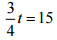(vi) 7m +7 = 77
(vii)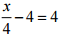(viii) 6y - 6 = 60
(ix)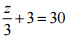Ques 5: Write the following equations in statement form:
(i) p + 4 = 15
(ii)  m - 7 = 3
(iii) 2m = 7
(iv) m/5 = 3
(v) 3m/5 = 6
(vi) 3p + 4 = 25
(vii) 4p - 2 = 18
(viii)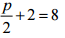Ans: (i) The sum of numbers p and 4 is 15.
(ii) 7 subtracted from m is 3.
(iii) Two times m is 7.
(iv) The number m is divided by 5 gives 3.
(v) Three-fifth of the number m is 6.
(vi) Three times p plus 4 gets 25.
(vii) If you take away 2 from 4 times p, you get 18.
(viii) If you added 2 to half is p, you get 8.

Ques 6: Set up an equation in the following cases:
(i) Irfan says that he has 7 marbles more than five times the marbles Parmit has. Irfan has 37 marbles. (Take m to be the number of Parmit’s marbles.)
(ii) Laxmi’s father is 49 years old. He is 4 years older than three times Laxmi’s age. (Take Laxmi’s age to be y years.)
(iii) The teacher tells the class that the highest marks obtained by a student in her class are twice the lowest marks plus 7. The highest score is 87. (Take the lowest score to be l
(iv) In an isosceles triangle, the vertex angle is twice either base angle. (Let the base angle be b in degrees. Remember that the sum of angles of a triangle is 180o
Ans: (i) Let m be the number of Permit's marbles.
∴ 5m + 7 = 37
(ii) Let the age of Laxmi be y years.
∴  3y + 4 = 49
(ii) Let the lowest score be l.
∴  2l + 7 = 87
(iv) Let the base angle of the isosceles triangle be b, so vertex angle = 2b.
∴  2b+b+b= 180°
⇒ 46 = 180°  [Angle sum property of a Δ]

Exercise 4.2
Ques 1: Give first the step you will use to separate the variable and then solve the equations:
(a) x - 1 = 0
(b) x + 1 = 0
(c) x + 1 =5
(d) x + 6 = 2
(e) y - 4 = -7
(f) y - 4 = 4
(g) y + 4 = 4
(h) y + 4 = -4
Ans: (a) x - 1 =0
⇒ x - 1 + 1 = 0 + 1  [Adding 1 both sides]
⇒  x = 1
(b) x + 1 = 0
⇒  x + 1 - 1 = 0 - 1   [Subtracting 1 both sides]
⇒  x = -1
(c)  x - 1 = 5
⇒  x - 1 + 1 = 5 + 1  [Adding 1 both sides]
⇒ x = 6
(d)  x + 6 = 2
⇒  x + 6 - 6 = 2 - 6  [Subtracting 6 both sides]
⇒ x = - 4
(e)  y - 4 = - 1
⇒ y - 4 + 4 = -7 +  4  [Adding4 both sides]
⇒  y = -3
(f)  y-4 = 4
⇒  y-4 + 4 = 4 + 4  [Adding 4 both sides]
⇒  y = 8
(g) y + 4 = 4
⇒  y + 4 - 4 = 4 - 4  [Subtracting 4 both sides]
⇒  y = 0
(h) y + 4 = -4
⇒  y + 4 - 4 = - 4 - 4  [Subtracting 4 both sides]
⇒  y = -8

Ques 2: Give first the step you will use to separate the variable and then solve the equations
(a) 3l = 42
(b) b/2 = 6
(c) p/7 = 4
(d) 4x = 25
(e) 8y = 36
(f) z/3 = 5/4
(g) a/5 = 7/15
(h) 20t = -10
Ans: (a) 3l = 42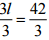[Dividing both sides by 3]
⇒ l = 14
(b) b/2 = 6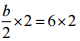[Dividing both sides by 3]
⇒ b = 12

(c) p/7 = 4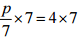[Dividing both sides by 2]
⇒ p = 28
(d) 4x = 25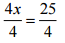[Dividing both sides by 7]
⇒ x = 25/4
(e) 8y = 36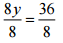[Dividing both sides by 4]
⇒ y = 9/2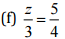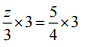[Dividing both sides by 8]
⇒ z = 15/4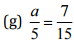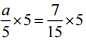[Dividing both sides by 3]
⇒ a = 7/3
(h) 20t = -10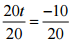[Dividing both sides by 5]
⇒ t = -1/2

Ques 3: Give first the step you will use to separate the variable and then solve the equations
(a) 3n - 2 = 46
(b) 5m + 7 = 17
(c) 20p/3 =40
(d) 3p/10 = 6
Ans: (a) 3n - 2 = 46
Step l: 3n-2+2 = 46 + 2
⇒ 3n = 48   [Adding 2 both sides]
Step II: 3n/3 = 48/3
⇒ n = 16    [Dividing both sides by 3]

(b) 5m+7 = 17
Step l: 5m + 7-7 = 17-7
⇒ 5m = 10     [Subtracting 7 both sides]
Step II:  5m/5 = 10/5
⇒ m = 2    [ Dividing both sides by 5]

(c) 20p/3 = 40
Step I: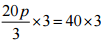[Multiplying both sides by 3]
⇒ 20p = 120
Step II: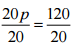[Dividing both sides by 20]
⇒ p = 6

(d) 3p/10 = 6
Step I: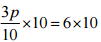[Multiplying both sides by 10]
⇒ 3p = 60
Step II: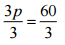[Dividing both sides by 3]
⇒ p = 20

Ques 4: Solve the following equation:
(a) 10p = 100
(b) 10p + 10 = 100
(c) p/4 = 5
(d) -p/3 = 5
(e) 3p/4 = 6
(f) 3s = -9
(g) 3s + 12 = 0
(h) 3s = 0
(i) 2q = 6
(j) 2q - 6 = 0
(k) 2q + 6 = 0
(l) 2q + 6 = 12
Ans:
(a) 10p = 100
⇒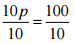[Dividing both sides by 10]
⇒ p = 10

(b) 10p+10 = 100
⇒  10p + 10-10 = 100-10    [Subtracting both sides 10]
⇒ 10 p = 90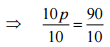[Dividing both sides by 10]
⇒ p = 9

(c) p/4 = 5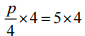[Multiplying both sides by 4]
⇒ p = 20

(d) -p/3 = 5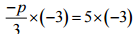[Multiplying both sides by - 3]
⇒ p = -15

(e) 3p/4 = 6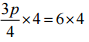[Multiplying both sides by 4]
⇒ 3p = 24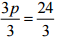[Dividing both sides by 3]
⇒ p = 8

(f) 3s = -9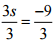[Dividing both sides by 3]
⇒ s = -3

(g) 3s + 12 = 0
⇒ 3s + 12 - 12 = 0 - 12  [Subtracting both sides 10]
⇒ 3s = -12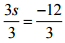[Dividing both sides by 3]
⇒ s = -4

(h) 3s = 0
⇒ 3s/3 = 0/3
⇒ 3s/3 = 0/3 [Dividing both sides by 3]
⇒ s = 0

(i) 2q = 6
⇒ 2q/2 = 6/2 [Dividing both sides by 2]
⇒ q = 3

(j) 2q - 6 = 0
⇒ 2q - 6 + = 0+6 [Adding both sides 6]
⇒ 2q = 6
⇒ 2q/2 = 6/2  [Dividing both sides by 2]
⇒ q = 3

(k) 2q + 6 = 0
⇒ 2q + 6 - 6 = 0-6 [Subtracting both sides 6]
⇒ 2q = -6
⇒ 2q/2 = -6/2 [Dividing both sides by 2]
⇒ q = -3

(l) 2q + 6 = 12
⇒ 2q + - 6 = 12 - 6  [Subtracting both sides 6]
⇒ 2q = 6
⇒ 2q/2 = 6/2  [Dividing both sides by 2]
⇒ q = 3

Offer running on EduRev: Apply code STAYHOME200 to get INR 200 off on our premium plan EduRev Infinity!

## Mathematics (Maths) Class 7

209 videos|109 docs|45 tests

,

,

,

,

,

,

,

,

,

,

,

,

,

,

,

,

,

,

,

,

,

;